## 20150421

### Major minor teatime diner

We’ve seen in a previous post that a musical phrase inversion in the major scale is guaranteed to remain within the major scale if and only if each note in the phrase is numerically subtracted from the mediant note of the scale. And we’ve seen that to achieve the same guarantee in the minor scale, the subtraction must be done from its subtonic.

Thus, for example, to invert the following phrase in the key of F#m: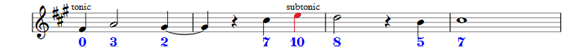The (blue) numbers underneath the notes are not figured-bass – they’re too big and too colourful for that – but are the numerical values of the notes within the scale they are embedded. Thus F# is note number zero (aka the tonic note) in the F#m key, and you will find the subtonic E of that scale (marked in red) ten semitones up.

Since the subtonic of the minor scale acts as the ‘inversion rail’ (we’re deprecating both the phrase and idea of ‘pitch axis’ and are replacing it with something of utility) from which all notes of the phrase are to be subtracted, we can see that – from left to right – the inverted phrase will begin on the subtonic (10 – 0 = 10), will drop three semitones down to the dominant (10 – 3 = 7), will then rise a semitone to the submediant (10 – 2 = 8) … etc and will end on the mediant (10 – 7 = 3):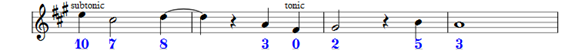Subtraction is simpler than reflection because you need no longer traverse the whole phrase from beginning to end, tracking the changes in direction in your first phrase and making, correspondingly, the exact opposite moves in your new phrase. Instead, you take each note on its own (start anywhere you like, jump around) and simply subtract its value from 10. There’s no need to remember where you were, so that you add or subtract some number dependent on that - you simply need to know where you are, and always subtract from 10. If your notes were in a minor key, they’ll stay there. Guaranteed.

If you use a clock face to represent all twelve chromatic notes, within which the seven notes of the minor scale are embedded, it’s relatively straightforward to see why subtractions from 10 yield inversions which remain in the same minor key.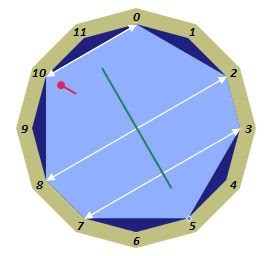minor inversions: { 0 ↔ 10, 2 ↔ 8, 3 ↔ 7, 5 ↔ 5 }

The thick white lines represent the inversions. The tonic note 0 is taken to the subtonic 10, and vice versa (10–0=10 and 10–10=0). The supertonic 2 is taken to the submediant 8 (10-2=8, 10-8=2), the mediant 3 to the dominant 7 (and vice versa) and the subdominant 5 (necessarily, shown with a very short white line) remains unaltered.

Now, grasp the big blue heptagonal knob (consider the white lines as being gouged into the polygon) and turn it 3 clicks anticlockwise. After that, you’re here: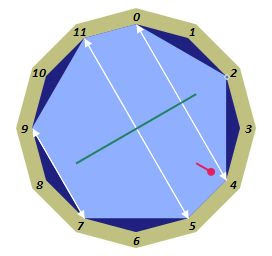major inversions: { 0 ↔ 4, 2 ↔ 2, 5 ↔ 11, 7 ↔ 9 }

Congratulations. We have just switched into the major scale.

The tonic note of the scale, 0, is always - necessarily - at the top. The knob is engineered so that one of the heptagonal vertices is pointing at zero, so there are only seven distinct positions and not the twelve you might think.

The subdominant (5) and dominant (7) happen to be in the same place, but the mediant (3), submediant (8) and subtonic (10) have each moved up a notch, to 4, 9 and 11, respectively. Which is of course exactly what you’d expect when switching from minor to major. What were the minor third, minor sixth and minor seventh become the (major) third, (major) sixth and (major) seventh (except you don’t usually bother to say ‘major’). The three ‘flattenings’ required to take us from any major key to the minor of the same name are evident here.

It should also be clear (from whichever gouged white line connects the tonic to its inversion rail) why inverting a phrase written in a major scale requires that you subtract each note of the original phrase from 4.

Just to show that the ‘knob’ view works, we can re-present our original phrase (at the beginning of this post) as one in the key of A major. The only difference is our blue annotations numbers under each note, which will now be the note numbers found in a major key.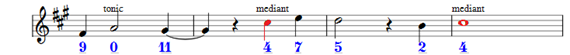phrase now interpreted as being in A

This time we’ve reddened the ‘inversion rail’ required for the major scale, which is the scale’s mediant (which in the key of A major is the C#). As we know, the mediant in a major key is the III (in roman numeral notation), the major third, and is a 4 semitone interval above the tonic.

These are exactly the same notes as before. Nothing has changed. The phrase is simply being re-viewed as one in a major key (necessarily the one corresponding to its earlier, relative minor, re-shown here for comparison)phrase originally interpreted as being in F#m

Inverting it, by subtracting every note from the value 4 (and adding 12, wherever necessary to re-notate it as a positive note number) gives us the new phrase: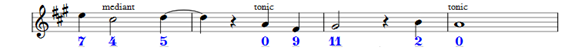Compare it with the earlier inversion, where the original was considered as being in a minor key:Again, exactly the same notes as the inversion before.

At this point, we remember our old Greek modes, and that the modern major and minor scales used to be known as, respectively, the Ionian and Aeolian modes. The following diagram shows all seven (where each vertex of the heptagon gets a turn at pointing to the zero) knobby orientations, each of which corresponds to one of the modes.

dor ion loc aeo mix lyd phr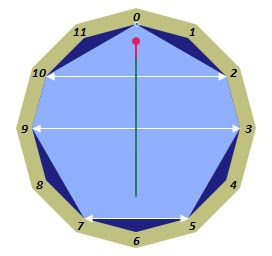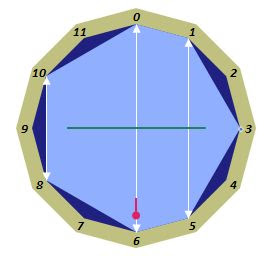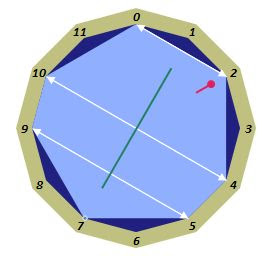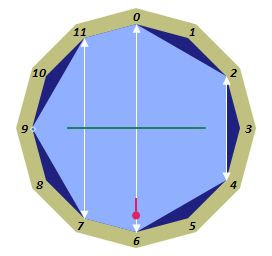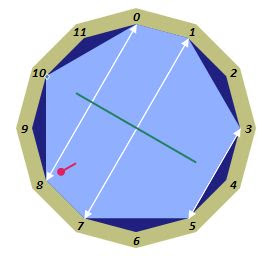Abbreviations should be obvious, but here they are anyway – ion=Ionian, dor=Dorian, phr=Phrygian, lyd=Lydian, mix=Mixolydian, aeo=Aeolian, loc=Locrian.

If you traverse the edges of each of these polygonal orientations clockwise from 0, and write down a 2 for a whole tone skip and a 1 for a semitone skip (almost, but not quite, the lengths of the polygonal edges clockwise from the top), you may represent the seven orientations/modes as dor=2122212, ion=2212221, loc=1221222, aeo=2122122, mix=2212212, lyd=2221221 and phr=1222122.

These are the seven possible permutations of a permutation group, typically notated with parentheses as, for example, (2212221) – where the parentheses contain a string of digits allowed to ‘rotate’ inside the parentheses by moving the last digit from the end to the beginning, or vice versa. E.g. take the final 1 of the 2212221 inside the parentheses and move it to the first position and you get (1221222). Which represents a rotation of the knob (clockwise) from an ionian to a locrian orientation.

It may be slightly surprising that it’s the Dorian mode which most clearly shows the vertical symmetry of the modern seven note diatonic scale, but it must be that way because it’s only the inversions of dorian phrases (intended to remain dorian) which subtract from the tonic (or 0). The short white line has to be there.

It’s an accident of symmetry, first in that the heptagon is symmetric at all (it need not have been) and second in that there’s no law of the universe which says that the 0 direction is ‘up’.

So it can surely be no accident that the Ionian (the modern major) and the Aeolian (the modern minor) appear to be set at two o’clock and five o’clock. Representing, as they do, the twin pinnacles of civilised society of maximal satisfaction and self-assuredness just after lunch and the more sober contemplation of the approaching teatime.